# Payback Period

Period of time required to recoup the cost of investment

## What is the Payback Period?

The Payback Period shows how long it takes for a business to recoup its investment. This type of analysis allows firms to compare alternative investment opportunities and decide on a project that returns its investment in the shortest time, if that criteria is important to them.

For example, a firm may decide to invest in an asset with an initial cost of \$1 million. Over the next five years, the firm then receives positive cash flows that diminish over time. When is the payback period? As seen from the graph below, the initial investment is fully offset by positive cash flows somewhere between periods 2 and 3.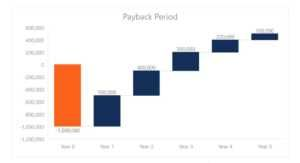### Payback Period Formula

To find exactly when this occurs, the following formula can be used: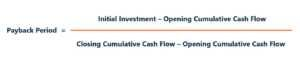Applying the formula to the example, we take the initial investment at its absolute value. The opening and closing period cumulative cash flows are \$900,000 and \$1,200,000, respectively. This is because as we noted, the initial investment is recouped somewhere between periods 2 and 3. Applying the formula provides the following: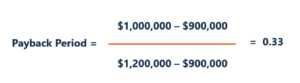As such, the payback period for this project is 2.33 years. The decision rule using the payback period is to minimize the time taken for the return of investment.

### Using the Payback Method

In essence, the payback period is used very similarly to a Breakeven Analysis but instead of the number of units to cover fixed costs, it considers the amount of time required to return the investment.

Given its nature, the payback period is often used as an initial analysis that can be understood without much technical knowledge. It is easy to calculate and is often referred to as the “back of the envelope” calculation. Also, it is a simple measure of risk as it shows how quickly money can be returned from an investment. However, there are additional considerations that should be taken into account when performing the capital budgeting process.

### Drawback 1: Profitability

While the payback period shows us how long it takes for the return of investment, it does not show what the return on investment is. Referring to our example, cash flows continue beyond period 3 but they are not relevant in accordance with the decision rule in the payback method.

Building on the previous example, the firm may have a second option of investing in another project that offers the following cash flows: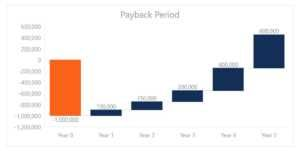The project would give a payback period of 4.25 years but generate higher returns on investment than the first project. However, based on the payback method, the firm would select the first project over this alternative. The implications of this are that the firm may choose investments with shorter payback periods at the expense of profitability.

### Drawback 2: Risk and the Time Value of Money

Another issue with the payback period is that it does explicitly discount for the risk and opportunity costs associated with the project. In some ways, a shorter payback period suggests lower risk exposure as the investment is returned at an earlier date. However, different projects may have exposure to different levels of risk even in the same period. Project risk is often determined by estimating WACC.

### Internal Rate of Return (IRR)

As an alternative to looking at how quickly an investment is paid back, and given the drawback outline above, it may be better for firms to look at the internal rate of return (IRR) when comparing projects.

Financial analysts will perform financial modeling and IRR analysis to compare the attractiveness of different projects.  By forecasting free cash flows into the future it is then possible to use the XIRR function in Excel to determine what discount rate sets the Net Present Value of the project to zero (the definition of IRR).

Since IRR does not take into account risk, it should be looked at in conjunction with payback period to determine which project is most attractive.

As you can see in the example below, a DCF model is used to graph the payback period (middle graph below).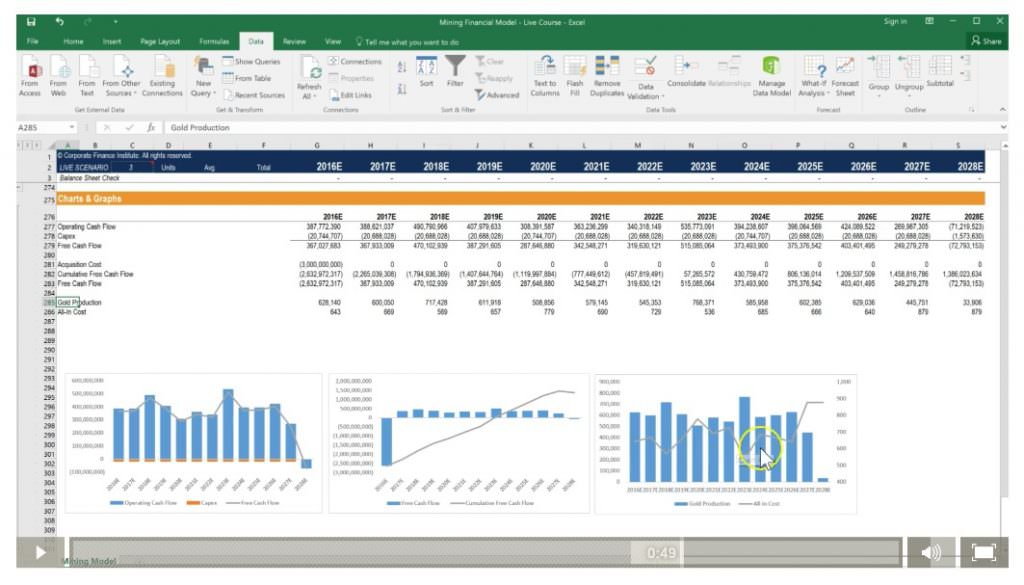Source: CFI Financial Modeling Courses online.